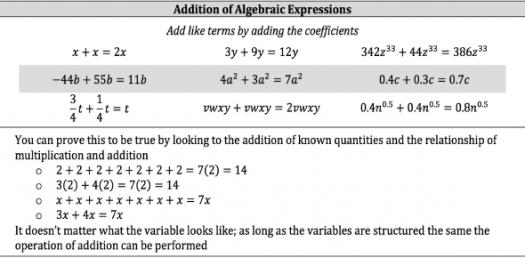# Algebraic Expressions

Approved & Edited by ProProfs Editorial Team
The editorial team at ProProfs Quizzes consists of a select group of subject experts, trivia writers, and quiz masters who have authored over 10,000 quizzes taken by more than 100 million users. This team includes our in-house seasoned quiz moderators and subject matter experts. Our editorial experts, spread across the world, are rigorously trained using our comprehensive guidelines to ensure that you receive the highest quality quizzes.
| By Nnorgardnorgard
N
Nnorgardnorgard
Community Contributor
Quizzes Created: 1 | Total Attempts: 5,382
Questions: 12 | Attempts: 5,418SettingsThis quiz is going to be about Algebraic Expressions. You will answer a few short answer questions. Good luck and remember to check over after you have finished!

• 1.

### 4 times a number plus 5 times the same number. (Use y)

Explanation
The given expression represents the sum of 4 times a number and 5 times the same number. By combining like terms, we can simplify the expression to 9y, which represents the total value obtained by adding 4y and 5y together.

Rate this question:

• 2.

### 7 more than one third of a number

Explanation
The given expression, 7+1/3x, represents the sum of 7 and one third of a number x. This can be understood by breaking down the expression: 1/3x represents one third of the number x, and when we add 7 to it, we get the result of 7 more than one third of the number x.

Rate this question:

• 3.

### The sum of 20 and a number divided by 5

Explanation
The given expression represents the sum of 20 and a number y, divided by 5. This can be simplified as (20+y)/5.

Rate this question:

• 4.

### Write in words 8/y (fraction)

Explanation
The given expression "8/y" represents the division of the number 8 by another number, denoted as "y". The answer is "eight divided by a number" because it describes the operation of dividing 8 by an unknown value, which is represented by the variable "y".

Rate this question:

• 5.

### A+b

Explanation
The given question states "a+b" and describes it as "A number increased by a number." This implies that the expression "a+b" represents the sum of two numbers, where one number is added to another number. Therefore, the correct answer is "A number increased by a number."

Rate this question:

• 6.

### Six more than a number

Explanation
The given expression "y+6" represents the phrase "six more than a number". This means that a certain number, represented by "y", is being added to 6. So, the expression "y+6" correctly represents the phrase "six more than a number".

Rate this question:

• 7.

### The product of 8 and a number

Explanation
The given expression represents the product of 8 and a number, which can be represented as 8y. The variable "y" represents the unknown number being multiplied by 8. Therefore, the correct answer is 8y.

Rate this question:

• 8.

### Twenty less a number

Explanation
The expression "20-y" represents the phrase "twenty less a number." The variable "y" represents the unknown number that is being subtracted from 20. Therefore, the expression "20-y" correctly represents the given phrase.

Rate this question:

• 9.

• 10.

### Five more than a number

Explanation
The given expression "5+y" represents the phrase "five more than a number". It implies that we are adding 5 to a certain number y, resulting in the sum of 5 and y. The expression does not simplify further as it is already in its simplest form.

Rate this question:

• 11.

### The difference between a number and ten

Explanation
The given expression (y-10) represents the difference between a number and ten. It subtracts 10 from the variable y, indicating that the value of y is being reduced by 10. This expression can be used to find the numerical difference between any number and ten by substituting the desired value for y.

Rate this question:

• 12.

### Product, sum, difference, and quotient are all answers that mean to put ( )s around the expression.

• A.

True

• B.

False

A. True
Explanation
The statement is true because product, sum, difference, and quotient are all mathematical operations that involve performing calculations on numbers. In order to clearly indicate the expression that needs to be calculated, it is common practice to put parentheses around it. Therefore, the answer is true as all these operations require the use of parentheses.

Rate this question:

Related TopicsBack to top